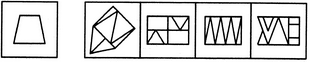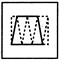# Non Verbal Reasoning - Embedded Images - Discussion

### Discussion :: Embedded Images - Section 1 (Q.No.25)

In each of the following questions, you are given a figure (X) followed by four alternative figures (1), (2), (3) and (4) such that figure (X) is embedded in one of them. Trace out the alternative figure which contains fig. (X) as its part.

25.

Find out the alternative figure which contains figure (X) as its part.(X)                (1)         (2)         (3)        (4)

 [A]. 1 [B]. 2 [C]. 3 [D]. 4

Explanation:Puneeth said: (Apr 4, 2018) It should be option A.

 Divya said: (Oct 17, 2020) Why not option A is the correct answer even we can find it in (1)?

 Aayush... said: (Oct 29, 2020) I think the answer is (D).